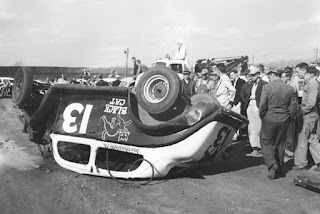- Why The number 13 is generally considered to be an unlucky number?
>>>>>>>>>>>>>>>>>>>>>>

# Why The number 13 is generally considered to be an unlucky number?The number 13 is considered an unlucky number in some countries.The end of the Mayan calendar's 13th Baktun was superstitiously feared as a harbinger of the apocalyptic 2012 phenomenon.Fear of the number 13 has a specifically recognized phobia, Triskaidekaphobia, a word coined in 1911. The superstitious sufferers of triskaidekaphobia try to avoid bad luck by keeping away from anything numbered or labelled thirteen. As a result, companies and manufacturers use another way of numbering or labelling to avoid the number, with

hotels and tall buildings being conspicuous examples (thirteenth floor). It's also considered unlucky to have thirteen guests at a table. Friday the 13th has been considered an unlucky day
Why 13 is famous as Unlucky?
Alexander wanted to be a God and accordingly made a 13th statue on the place of his capital. He died and people considered that his will to be a 13th God (12 gods, one for each month were known) was the reason of his death. After that this number was considered as unlucky.
According to historians, there were 13 people at Christs Last Supper and Christ was crucified on Friday 13th. So, Friday 13th is considered as Unlucky.
On Friday 13th October 1307, King Philip IV of France ordered the arrest of the Knights Templar . The Templars were charged with numerous offences. Many of the accused confessed to these charges under torture. Many stories claim that the Friday the 13th superstition comes from the historical destruction of the Knights Templar.
What is so interesting about 13?
Leaving aside the superstitions views, let us see how fascinating the number 13 from mathematical point of view is.
• Reverse of the square of 13 is the same as the square of the reverse of 13 i.e. 132= 169; on reversing 169, we get 961 which is the same as the square of reverse of 13 i.e. 312 = 961.
13 x 13 = 169
31 x 31 = 961
• Number 13 is the smallest prime number which can be expressed as the sum of the squares of two prime numbers i.e.
13 = 22 + 32
Where 2, 3, 13 are prime numbers.
• From number 13 if we subtract the sum of its digits, we get a perfect square i.e.
13 - (1 + 3) = 9 = 32
Also if the product of digits of 13 is added to it, we again a perfect square i.e.
13 + (1 x 3) = 16 = 42
• Consider the equations:
132= 169
312= 961
If we insert the + sign between all the digits of above equation, the equation still holds good i.e.
(1+3)2 = 1+6 +9
(3+1)2 = 9 +6+1
• The smallest number whose sum of digits is 13 is a perfect square i.e. 49 = 72 and 4 + 9 = 13.
• The smallest square which contains last three digits alike is 1444. The sum of digits of this number is also 13 i.e.
1+4+ 4+ 4 = 13
• If we place the number 13 before its reverse i.e. 31 we get the number 1331 which is a cubic number i.e. 1331= 113
• If we take the cube of number 13 i.e. 133 = 2197 and rearrange the digits of the cube 2197 we get the famous Ramanujan's number 1729.
• 13 is the average number of the prime factors of the famous Ramanujan's number 1729. i.e.
1729 7 x 13 x 19
(7 + 13 + 19) ÷ =13
• Take the square of the number 13 i.e. 132 = 169. Now we see that 169 contains many perfect squares in it:

The sum of the digits of the number 169 is a perfect squares and is the square of the sum of the digits of the original number i.e.
1+6+9 = 16 = 42 =(1+3)2
Divide the number in two blocks i.e. 16 and 9. We see that both are perfect square numbers and their sum and product is a perfect square:
16 + 9 = 25 = 52
16 x 9 = 144 = 122
The number 169 which is perfect square can be expressed as sum of two square i.e.
169 = 132 = 52 + 122
The number 169 can be expressed by number of its two block i.e. 16 and 9 which are perfect squares as follows:
169 = (16 + 9) + (16 x 9)
• Sum of the numbers from 1 to 13 gives 91 which is the smallest number which can be expressed as the sum of two cubes and also as the difference of two cubes i.e.
91 = 33 + 43
91 = 63 - 53
What is the effect of such superstitions?
Some people won't go to work on Friday the 13th.
Some people won't eat in restaurants on Friday the 13th
Some people may not like wedding on Friday the 13th.
Some people don't like to travel on Friday the 13th.
Hospital beds, operating rooms and maternity units have avoided numbering rooms with the number 13.
Its not unusual to find auditorium and stadium seats where number 13 is missing. Even airlines and theaters avoid using the number thirteen.Many buildings don't have 13th floor. Many hotels don't have a room with number 13. It is sometimes called as 12A. Because superstitious people avoid themselves to be admitted into a hotel room with the number 13 or on the 13th floor of buildings.
According to superstition, any one with 13 letters in their name is regarded to have Devil's luck.
Brussels Airlines even had to change its logo after superstitious passengers complained quite strongly about the 13 dots that were used to create the letter "B" in the Brussels Airlines logo. They actually changed the logo to meet consumer needs.